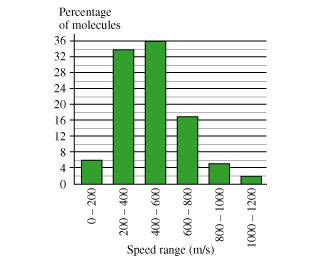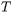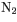# Problem: The kinetic theory of gases states that the kinetic energy of a gas is directly proportional to the temperature of the gas. A relationship between the microscopic properties of the gas molecules and the macroscopic properties of the gas can be derived using the following assumptions: The gas is composed of pointlike particles separated by comparatively large distances. The gas molecules are in continual random motion with collisions being perfectly elastic. The gas molecules exert no long-range forces on each other. One of the most important microscopic properties of gas molecules is velocity. There are several different ways to describe statistically the average velocity of a molecule in a gas. The most obvious measure is the average velocity Vavg. However, since the molecules in a gas are moving in random directions, the average velocity is approximately zero. Another measure of velocity is (V^2)avg, the average squared velocity. Since the square of velocity is always positive, this measure does not average to zero over the entire gas. A third measure is the root-mean-square (rms) speed,Vrms , equal to the square root of (V^2)avg. The rms speed is a good approximation of the the typical speed of the molecules in a gas. This histogram (Figure 1) shows a theoretical distribution of speeds of molecules in a sample of nitrogen () gas. In this problem, you'll use the histogram to compute properties of the gas.What is the temperatureof the sample ofgas described in the histogram?Express your answer in degrees Celsius to three significant figures.

###### FREE Expert Solution

Root mean square expression:

$\overline{){{\mathbf{v}}}_{\mathbf{r}\mathbf{m}\mathbf{s}}{\mathbf{=}}\sqrt{\frac{\mathbf{3}\mathbf{R}\mathbf{T}}{\mathbf{m}}}}$

vrms = 518.8 m/s

R = 8.314 J/mol•K###### Problem DetailsThe kinetic theory of gases states that the kinetic energy of a gas is directly proportional to the temperature of the gas. A relationship between the microscopic properties of the gas molecules and the macroscopic properties of the gas can be derived using the following assumptions: The gas is composed of pointlike particles separated by comparatively large distances. The gas molecules are in continual random motion with collisions being perfectly elastic. The gas molecules exert no long-range forces on each other. One of the most important microscopic properties of gas molecules is velocity. There are several different ways to describe statistically the average velocity of a molecule in a gas. The most obvious measure is the average velocity Vavg. However, since the molecules in a gas are moving in random directions, the average velocity is approximately zero. Another measure of velocity is (V^2)avg, the average squared velocity. Since the square of velocity is always positive, this measure does not average to zero over the entire gas. A third measure is the root-mean-square (rms) speed,Vrms , equal to the square root of (V^2)avg. The rms speed is a good approximation of the the typical speed of the molecules in a gas. This histogram (Figure 1) shows a theoretical distribution of speeds of molecules in a sample of nitrogen () gas. In this problem, you'll use the histogram to compute properties of the gas.

What is the temperatureof the sample ofgas described in the histogram?Express your answer in degrees Celsius to three significant figures.